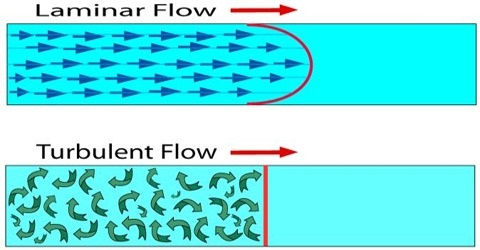# Different Types of Flow of Fluids

Flow of fluids can be of different types:

Flow of fluid from one place to another place is called flow of fluid. Fluid Flow is a part of fluid mechanics and deals with fluid dynamics. A fluid such as gases and liquids in motion is called as fluid flow. If a fluid is in-compressible and if there is no viscosity then it is called ideal fluid.

Fluid flow has all kinds of aspects — steady or unsteady, compressible or in-compressible, viscous or nonviscous, and rotational or irrotational, to name a few. Some of these characteristics reflect properties of the liquid itself, and others focus on how the fluid is moving.

(a) Uniform motion: If velocity always remains constant, then it is called uniform motion.

(b) Non-uniform motion: If velocity does not remain constant at all times, then it is called non-uniform motion.

(c) Steady motion: If the velocity all over the fluid remains same, then it is called steady motion. In steady fluid flow, the velocity of the fluid is constant at any point.

(d) Unsteady motion: If velocity all over the fluid does not remain same, then it b called unsteady motion. When the flow is unsteady, the fluid’s velocity can differ between any two points.

(e) Streamline motion: If different lavers of a fluid travel parallel to each other, then it is called streamline motion.

(f) Turbulent motion: If different lavers of a fluid do not travel parallel to each other, rather create turbulence and eddies then that motion is called turbulent motion.